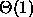CSE 5311 Spring 1998
Homework #1
Due: February 4, 1998

1. Design and analyze an iterative version of Sequential Search (SEARCH(A, k, p, r)) that returns the position of k in the sorted integer array A[pr]. If k is not found, the algorithm returns -1. For your algorithm perform the following specific tasks:

a.
Give pseudocode for your iterative SEARCH(A, k, p, r) algorithm. NOTE: You may not use global variables in your algorithm.

b.
Perform a line-by-line analysis of your algorithm and derive a precise expression T(n) for the running time, where n is the length of the array. You may assume that each line of pseudocode takes unit time (i.e.,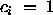).

c.
Describe the situation resulting in the best-case running time of your algorithm and derive an expression for T(n) in this case. Also give an asymptotically-tight expression for T(n).

d.
Describe the situation resulting in the worst-case running time of your algorithm and derive an expression for T(n) in this case. Also, give an asymptotically-tight expression for T(n).

2. Design and analyze a recursive version of Binary Search SEARCH(A, k, p, r) algorithm that uses a divide-and-conquer approach to perform a search for k (return the position of k in the array or return -1) in which the algorithm divides the sorted array in half and calls itself recusively on each half. For your algorithm perform the following specific tasks:

a.
Give pseudocode for your recursive SEARCH(A, k, p, r) algorithm. NOTE: You may not use global variables in your algorithm.

b.
Perform a line-by-line analysis of your algorithm. Describe the situations resulting in the best-case and worst-case performances of your algorithm.

c.
Derive a precise recurrence for T(n) in the worst case, and solve the recurrence.

3. The following chart shows the run time of algorithms that use f(n) operations to run on a computer where each opration takes one second to execute. Fill in the blanks in the chart.

 n f(n) = lg n f(n) = n f(n) = n lg n f(n) =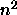f(n) =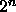10 2.3s 10s 23.0s 100s 1024s 20 50 100 1,000 10,000

4. For each of the following, answer ``yes'', ``no'', or ``cannot tell''. Explain your reasoning.

1. Is= O()?
2. Is=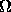()?
5. Arrange the following expressions by growth rate from slowest to fastest.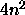n!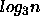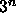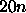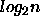6. Give asymptotically tight upper and lower bounds for T(n) in each of the following currences. Assume that T(n) is constant for n <= 2. For each problem use two of the described methods to solve the recurrence.

1. T(n) = 2T(n/2) +.
2. T(n) = 3T(n-2) +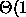3. T(n) = 3T(n/3) +